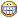In this tutorial, we will creat a random string, get a value from an array. Why do we need it? Simple, sometime, we have license that limit number of query to 90, but we need more, so we register 5 or more licenses, then we use PHP random from array to get 1 license. Or we want to creat a 9 capital, number value for license key (for whom need generate a license key).

## Demo: PHP Random String Generator, Random Value from Array

Now, let’s go.
First, we will generate a Random string:

```function rand_str(\$length = 32, \$chars = 'ABCDEFGHIJKLMNOPQRSTUVWXYZ1234567890')
{
\$chars_length = (strlen(\$chars) - 1);
\$string = \$chars{rand(0, \$chars_length)};
for (\$i = 1; \$i < \$length; \$i = strlen(\$string))
{
\$r = \$chars{rand(0, \$chars_length)
};
if (\$r != \$string{\$i - 1}) \$string .=  \$r;  }
return \$string;
}```

That function will generate a random string with capital character and number. Number of character will define by us.
Example:

`echo rand_str (\$length = 15, \$chars = 'ABCDEFGHKLMNPQRSTUVWXYZ123456789');`

Will generate a random string in 15 chracter length, from these character only: ABCDEFGHKLMNPQRSTUVWXYZ123456789
If you need lowercase character, just define it in \$chars. Something like this:

`echo rand_str (\$length = 15, \$chars = 'ABCDEFGHKLMNPQRSTUVWXYZabcdefghijklmnopqrstuvz123456789');`

Let’s try:

`\$licenselist = array('02AD-C7RC-ZLEH', '0298-ZACX-FF4F', '0378-34CX-FCVF', '6598-96CX-263F', '7246-823X-980F', '4358-9245-B678');`

Now, we get 1 license key from that array:

```function array_random(\$arr, \$num = 1) {
shuffle(\$arr);

\$r = array();
for (\$i = 0; \$i < \$num; \$i++) {
\$r[] = \$arr[\$i];
}
return \$num == 1 ? \$r : \$r;
}```

Used:

```\$license = array_random(\$licenselist);
Well, that’s it. Is it simple?